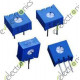200 Ohm 3386 Potentiometer Variable Code = 201 ..
1K Ohm 3386 Potentiometer VariableCode = 102 ..
2K Ohm 3386 Potentiometer VariableCode = 202 ..
5K Ohm 3386 Potentiometer Variable Code = 502 ..
10K Ohm 3386 Potentiometer Variable Code = 103 ..
20K Ohm 3386 Potentiometer VariableCode = 203 ..
50K Ohm 3386 Potentiometer VariableCode = 203 ..
100K Ohm 3386 Potentiometer Variable Code = 104 ..
200K Ohm 3386 Potentiometer VariableCode = 204 ..
500K Ohm 3386 Potentiometer VariableCode = 504 ..
1M Ohm 3386 Potentiometer Variable Code = 105 ..
Showing 1 to 11 of 11 (1 Pages)